# Scheduling

Operating System perform scheduling in the following simple ways:

• Dispatch orders immediately
• scheduling is simple FIFO (First-Come-First-Serve)
• Dispatch simple orders first
• maximize number of orders processed over time
• maximize throughput (SJF)
• Dispatch complex orders first
• maximize utilization of CPU, devices, memory

## CPU Scheduler

• Decides how and when process (and their threads) access shared CPUs
• Runs when
• CPU becomes idle
• timeslice expired timeout

Context switch, enter user mode, set PC and go! <= Thread is dispatched on CPU.

• Which task should be selected?
• Scheduling policy/algorithm
• How is this done?
• Depends on runqueue data structure

### "Run-to-completion" Scheduling

• Initial assumptions
• known execution time
• no preemption
• single CPU
• Metrics
• throughput
• average job completion time
• average job wait time
• CPU utilization

## Scheduling algorithms:

### 1. First Come First Serve (FCFS)

• Schedules tasks in order of arrival
``````runqueue = queue(FIFO)
``````

If T1, T2, T3 arrive in the given order and T1 has execution time 1s, T2 10s and T3 1s then :

• Throughput = 3/(1+10+1) = 3/12 = 0.25s
• Average completion time = (1 + 11 + 12)/3 = 8s
• Average wait time = (1+1+11)/3 = 4s

### 2. Shortest Job First (SJF)

• Schedules tasks in order of execution time
• Therefore for the above example, T1(1s) > T3(1s) > T2(10s)
``````runqueue = ordered(queue)

//or

runqueue = tree()
``````

For SJF,

• Throughput = 3/(1+10+1) = 3/12 = 0.25s
• Average completion time = (1 + 2 + 12)/3 = 5s
• Average wait time = (0+1+2)/3 = 1s

### Preemptive Scheduling

• SJF + Preemption

T2 arrives first.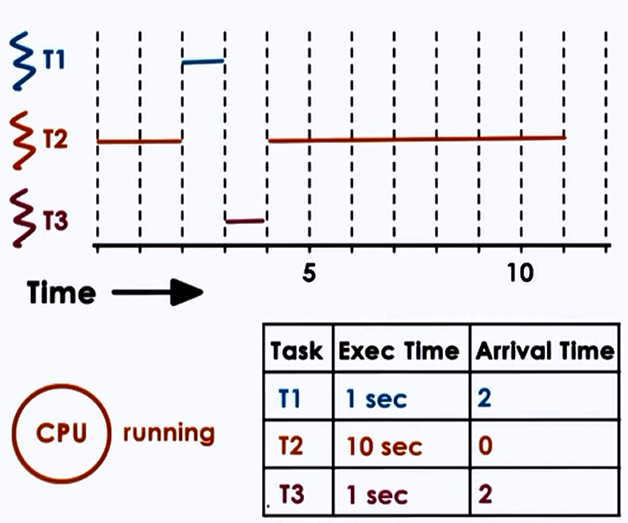### Priority Scheduling

• Tasks have different priority levels
• Run highest priority task next (preemption)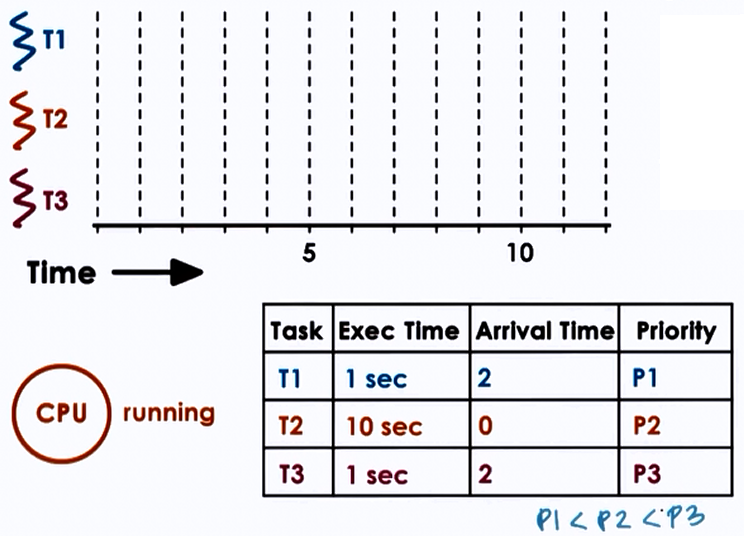``````runqueue = per priority_queue()

//or

runqueue = tree() ordered on priority
``````
• low priority task stuck in runqueue => starvation
• "priority aging"
• priority = f(actual priority, time spent in runqueue)
• prevents starvation

### 3. Round-Robin Scheduling

• Pick up the first task from queue (like FCFS)
• Task may yield to wait on I/O (unlike FCFCS)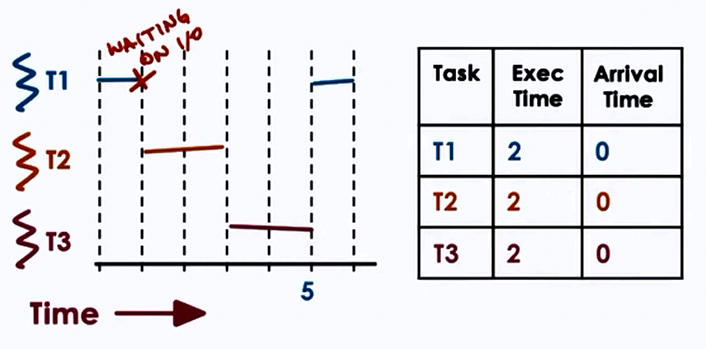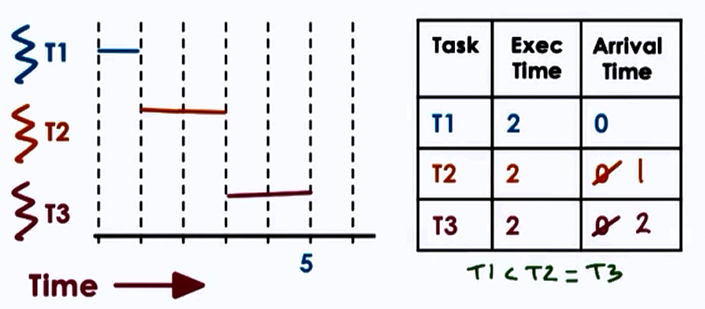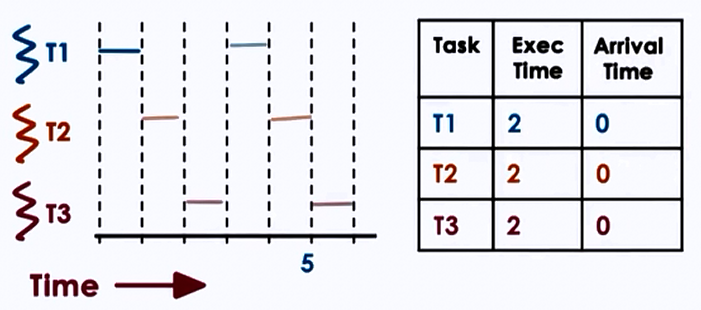#### Timeslicing

• Timeslice = max amount of uninterrupted time given to a task
• task may run less than timeslice
• has to wait on I/O sync
• will be placed on queue
• higher priority task becomes runnable
• using timeslice tasks are interleaved
• timesharing the CPU
• CPU bound tasks => preemption after timeslice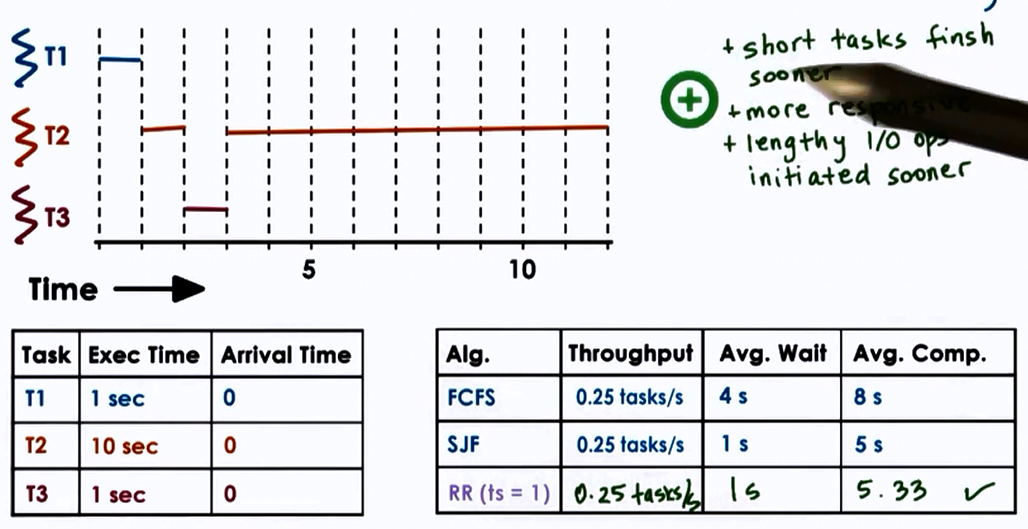• More responsive
• Lengthy I/O operations initiated sooner
• best to keep timeslice > context-switch-time

Disdvantages

#### How long should a timeslice be be?

• should balance benefits and overheads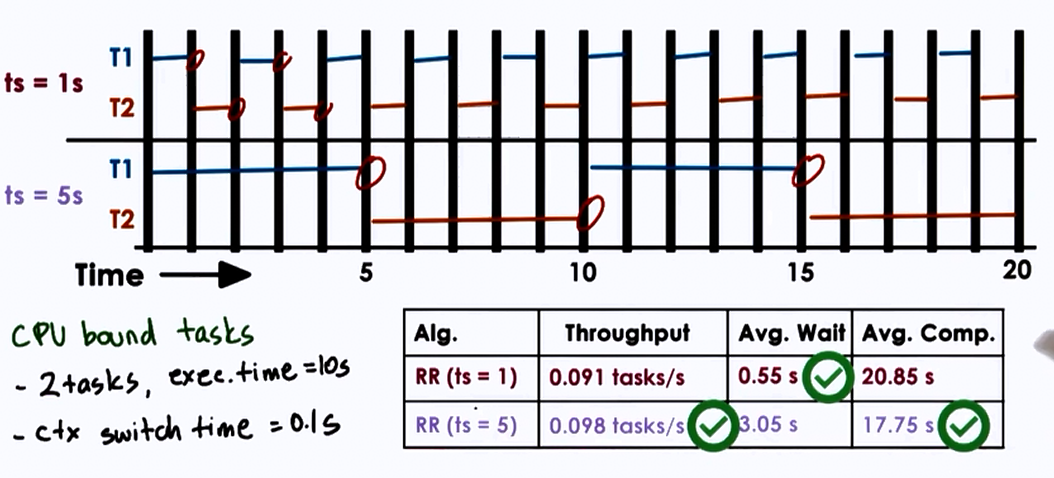• Hence, for CPU bound tasks, larger timeslice values are better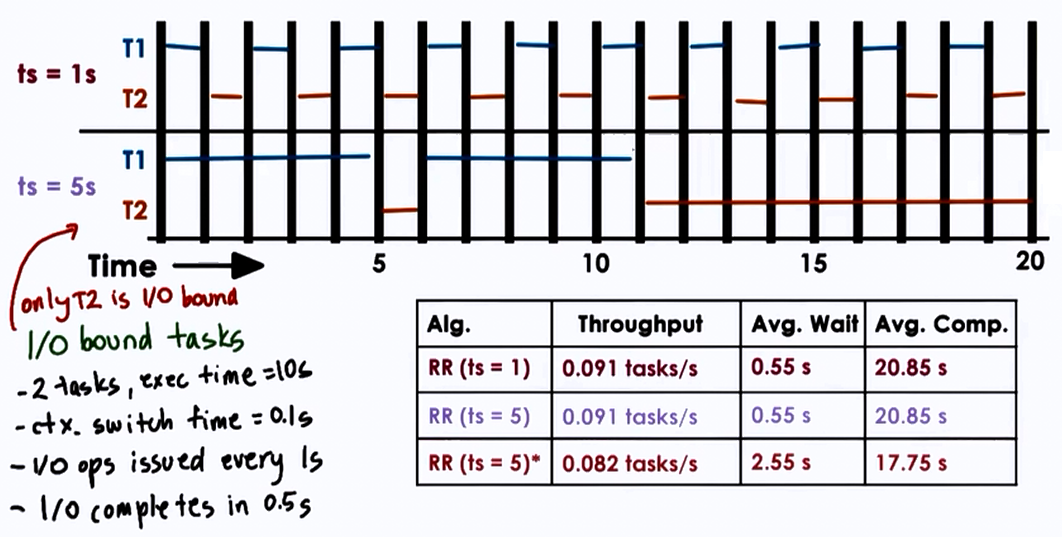• Hence, for I/O bound tasks, smaller timeslice values are better
• Keeps CPU and I/P devices busy, I/O bound tasks run quickly, makes I/O requests responds to a user.

### Summary

• CPU bound tasks prefer longer timeslices